# Logistic Regression in Machine Learning

## Logistic Regression in Machine Learning

In this article, I am going to discuss Logistic Regression in Machine Learning with Examples. Please read our previous article where we discussed Linear Regression in Machine Learning with Examples.

##### Logistic Regression in Machine Learning

Logistic regression is a classification technique that uses supervised learning to estimate the likelihood of a target variable. Because the nature of the target or dependent variable is dichotomous, there are only two viable classes. Simply said, the dependent variable is binary in nature, with data represented as either 1 (for success/yes) or 0 (for failure/no).

A logistic regression model predicts P(Y=1) as a function of X mathematically. It is one of the most basic ML techniques that may be used to solve a variety of classification issues such as spam identification, diabetes prediction, cancer diagnosis, and so on.

In general, logistic regression refers to binary logistic regression with binary target variables, but it may also predict two other types of target variables. Logistic regression may be classified into the following types based on the number of categories:

1. Binomial or binary: A dependent variable in this form of classification will have just two potential values: 1 or 0. These variables might, for example, indicate success or failure, yes or no, victory or loss, and so on.
2. Multinomial: The dependent variable in such a classification can have three or more alternative unordered categories or types with no quantitative significance. These variables may, for example, represent “Type A,” “Type B,” or “Type C.”
3. Ordinal: In this sort of categorization, the dependent variable might have three or more potential ordered categories or types with quantitative significance. For example, these variables may indicate “bad” or “good,” “very good,” or “Excellent,” with scores ranging from 0 to 2.
##### Assumptions for Logistic Regression in Machine Learning
• Before we dive into logistic regression implementation, we must be aware of the following assumptions regarding the same.
• The target variables in binary logistic regression must always be binary, and the intended outcome is indicated by factor level 1.
• The model should not have any multicollinearity, which indicates that the independent variables must be independent of one another.
• Our model must incorporate relevant variables.

### Case Study in Banking Domain

##### Introduction

According to the CMD’s most recent Quarterly Report on Household Debt and Credit, overall household debt climbed by \$ 92 billion in the third quarter of 2019 to \$ 13.95 trillion. It was the twenty-first straight quarterly gain, and the total is now \$ 1.3 trillion more than the previous peak of \$ 12.68 trillion in the third quarter of 2008. In the third quarter, non-housing balances climbed by \$ 64 billion, with increases across the board, including \$ 18 billion in auto loans, \$ 13 billion in credit card balances, and \$ 20 billion in student loans.

##### Problem Statement

IndNatBank is a peer-to-peer lending finance organization that lends to potential consumers all around India. They earn depending on the risk of the loans they offer to borrowers. They intend to estimate the risk of giving loans to new customers based on prior data, which will also assist to enhance the customization user experience while applying for loans.

They have skilled staff who apply sophisticated rules to give services to their clients. However, as the number of data grows, traditional methods of risk assessment may be detrimental to the firm. They want to automate their process so that the machine can discover patterns from their data and provide a better client experience.

##### Importing Libraries
```# For Panel Data Analysis
import pandas as pd
#from pandas_profiling import ProfileReport
pd.set_option('display.max_columns', None)
pd.set_option('display.max_rows', None)
pd.set_option('mode.chained_assignment', None)

# For Numerical Python
import numpy as np

# For Random seed values
from random import randint

# For Data Visualization
import matplotlib.pyplot as plt
%matplotlib inline
import seaborn as sns

# For Scientific Computation
from scipy import stats

# For Preprocessing & Scaling
from sklearn.preprocessing import LabelEncoder, OneHotEncoder, MinMaxScaler, StandardScaler

# For Feature Selection
from sklearn.feature_selection import SelectFromModel

# For Data Modeling and Evaluation
from sklearn.model_selection import train_test_split
from sklearn.linear_model import LogisticRegression
from sklearn.ensemble import RandomForestClassifier
from xgboost.sklearn import XGBClassifier

# For Machine Learning Model Evaluation
from sklearn.metrics import classification_report
from yellowbrick.classifier import PrecisionRecallCurve
from xgboost import to_graphviz, plot_importance

# To handle class imbalance problem
from imblearn.over_sampling import SMOTE

# To Disable Warnings
import warnings
warnings.filterwarnings(action = "ignore", message = '')

print('Data Shape:', data.shape)
```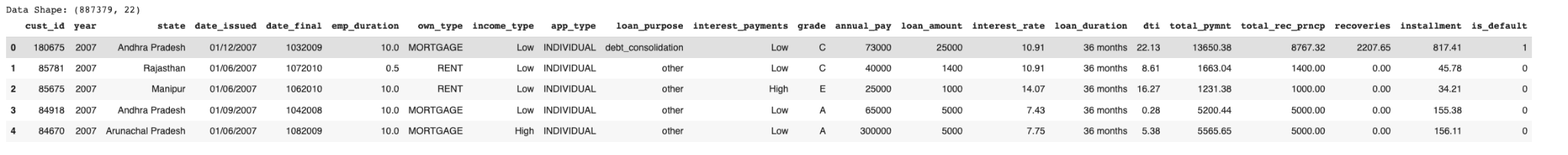##### Data Description

data.describe()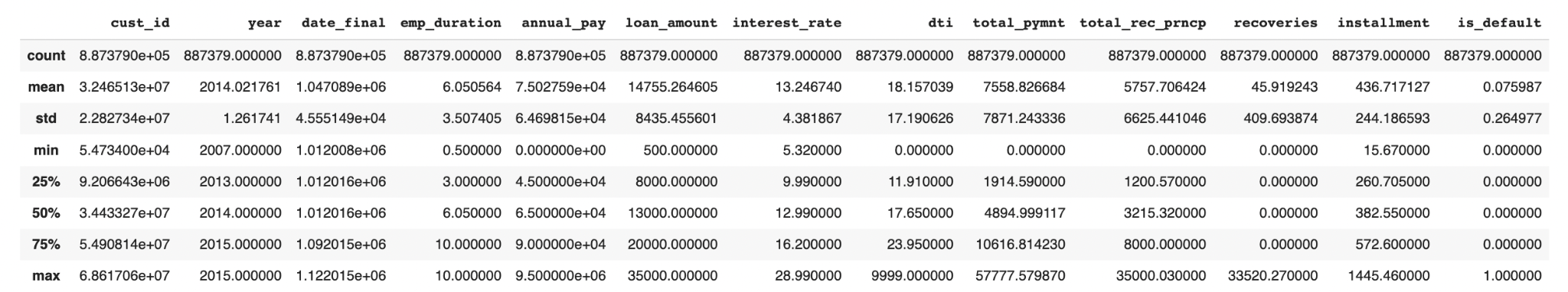data.info()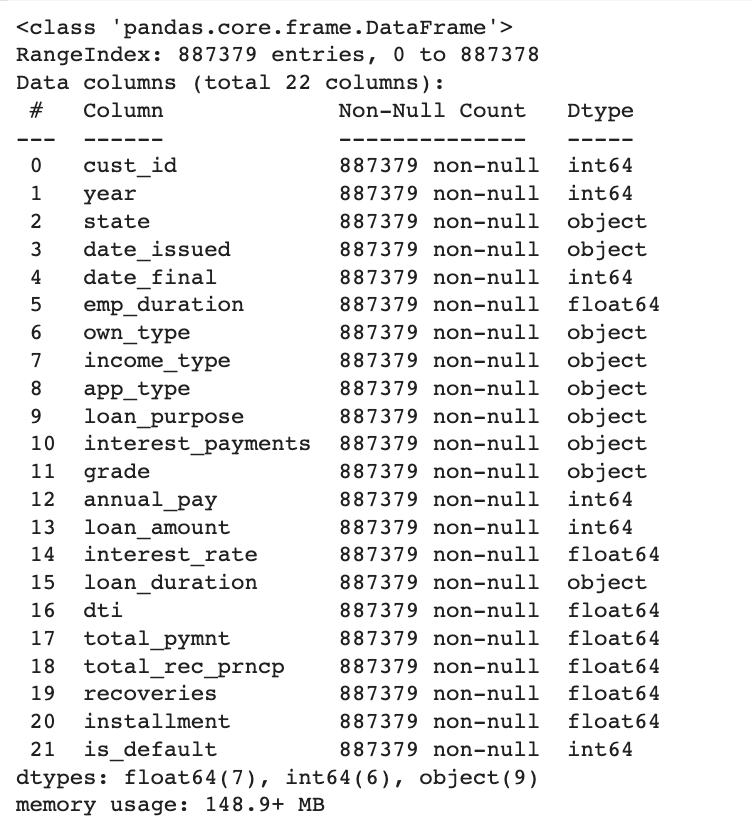##### Data Pre-Processing
```# Correcting types of features
data['date_issued'] = pd.to_datetime(data['date_issued'])
data['date_final'] = pd.to_datetime(data['date_final'], format = '%d%m%Y')
data['is_default'] = data['is_default'].astype(bool)

# Dropping cust_id as it is unique
data2 = data.copy()
data2 = data2.drop('cust_id', axis = 1)
```

#### EDA

##### Question 1: What is the proportion of customers who are defaulters and who are not?
```print('Customers who are not default:', data['is_default'].value_counts())
print('Customers who are default:', data['is_default'].value_counts())

space = np.ones(2)/10
data['is_default'].value_counts().plot(kind = 'pie', explode = space, fontsize = 14, autopct = '%3.1f%%', wedgeprops = dict(width=0.15),
shadow = True, startangle = 160, figsize = [13.66, 7.68], legend = True)
plt.legend(['Not Default', 'Default'])
plt.ylabel('Category')
plt.title('Proportion of default customers', size = 14)
plt.show()
```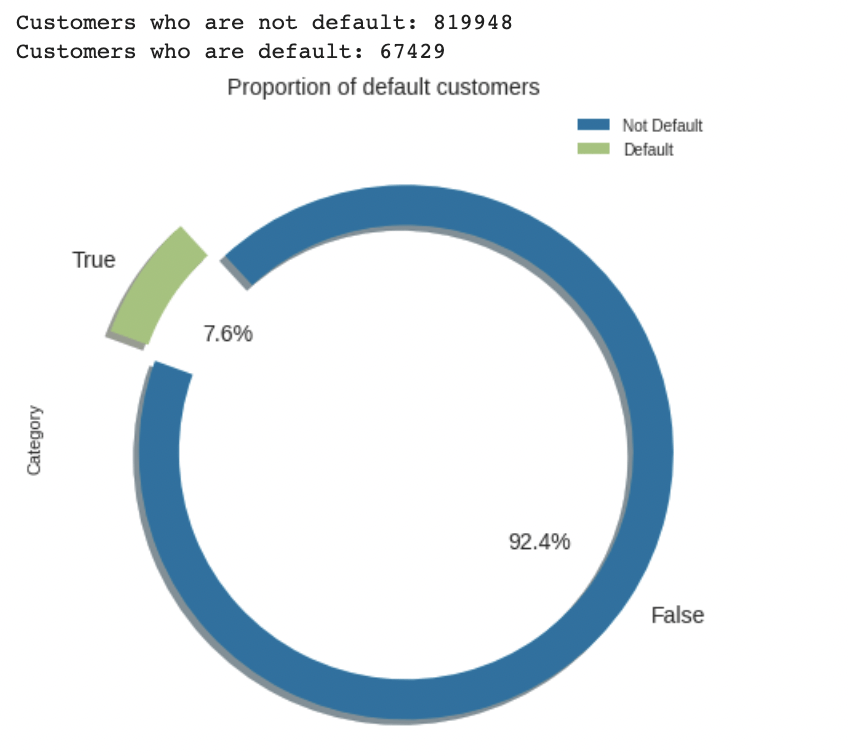##### Question 2: What is the rate of loan default with respect to final_date?
```figure = plt.figure(figsize = [15, 8])

data[data['is_default'] == 1]['date_final'].value_counts().plot(kind = 'line')

plt.xlabel('Year', size = 14)
plt.ylabel('Frequency', size = 14)
plt.title('Loan Default rate at each year', size = 16)
plt.show()
```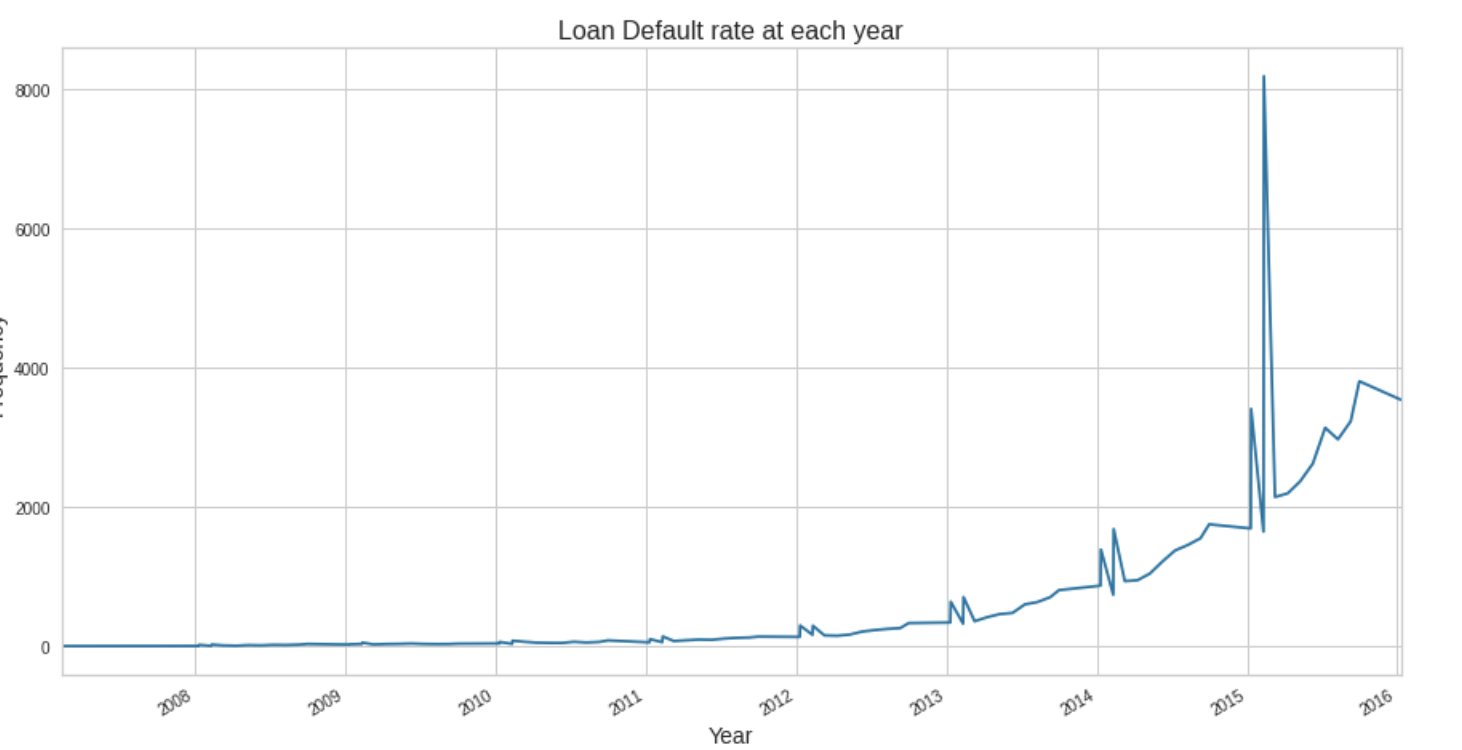##### Question 3: What is the frequency & proportion of ownership type that has been acquired with respect to the loan?
```print(data['own_type'].value_counts())

# Bar Plot
colors_list = ['lightcoral', 'lightgreen', 'mediumturquoise']
figure = plt.figure(figsize = [15, 8])
plt.subplot(1, 2, 1)
sns.barplot(data['own_type'].value_counts().index, data['own_type'].value_counts(), palette  = colors_list)
plt.yticks(range(0, 500000, 20000))
plt.xlabel('Ownership Type')
plt.ylabel('Frequency')
plt.title('Frequency occurence of Ownership Type', y=1.05, size = 14)

explode_list = [0, 0 , 0.2]

plt.subplot(1, 2, 2)
# Pie Plot
data['own_type'].value_counts().plot(kind = 'pie', figsize = [10, 5], autopct = '%1.1f%%', startangle = 90,
shadow = True, labels = None, pctdistance = 1.12, colors = colors_list,
explode = explode_list)
plt.title('Proportion of each Ownership Type w.r.t loan', y = 1.05, size = 14)
plt.ylabel('')
plt.axis('equal')
plt.legend(labels = data['own_type'].value_counts().index, loc = 'upper left', frameon = False)
plt.show()
```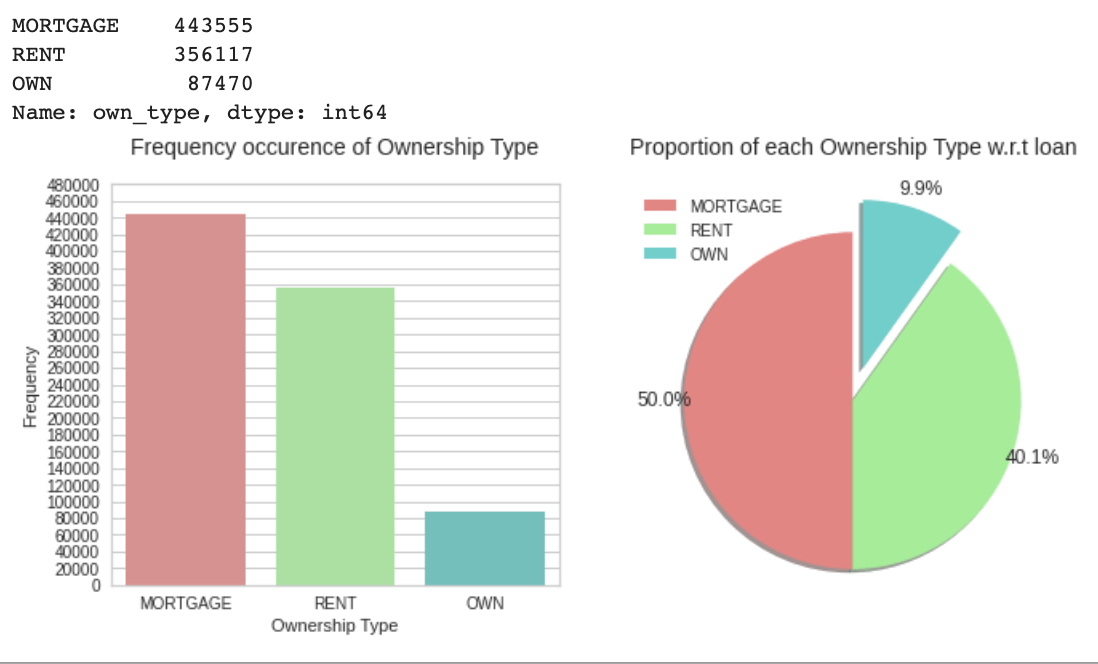data.drop(labels = [‘cust_id’, ‘date_issued’, ‘date_final’,’State’], axis = 1, inplace = True)

##### Label Encoding
```ordered_labels = ['year', 'income_type', 'app_type', 'interest_payments', 'grade', 'loan_duration']
encode = LabelEncoder()

for i in ordered_labels:
if isinstance(data[i].dtype, object):
data[i] = encode.fit_transform(data[i])

data = pd.get_dummies(data = data, columns = ['own_type', 'loan_purpose'])
print('Data Shape:', data.shape)

std_scale = StandardScaler()
scale_fit = std_scale.fit_transform(X)

X_data = pd.DataFrame(scale_fit, columns = X.columns)

X_train, X_test, y_train, y_test = train_test_split(X_data, y, test_size = 0.2, random_state = 42, stratify = y)
print('Train Shape:', X_train.shape, y_train.shape)
print('Test Shape:', X_test.shape, y_test.shape)
```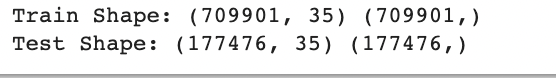##### Model Building
```log  = LogisticRegression(random_state = 42)
log.fit(X_train, y_train)
y_pred = log.predict(X_test)

# Accuracy Estimation
print('Accuracy Score (Train Data):', np.round(log.score(X_train, y_train), decimals = 3))
print('Accuracy Score (Test Data):', np.round(log.score(X_test, y_test), decimals = 3))

# Classification Report
logistic_report = classification_report(y_test, y_pred)
print(logistic_report)

# Precision Recall Curve
figure = plt.figure(figsize = [10, 8])
PRCurve(model = log)
plt.show()```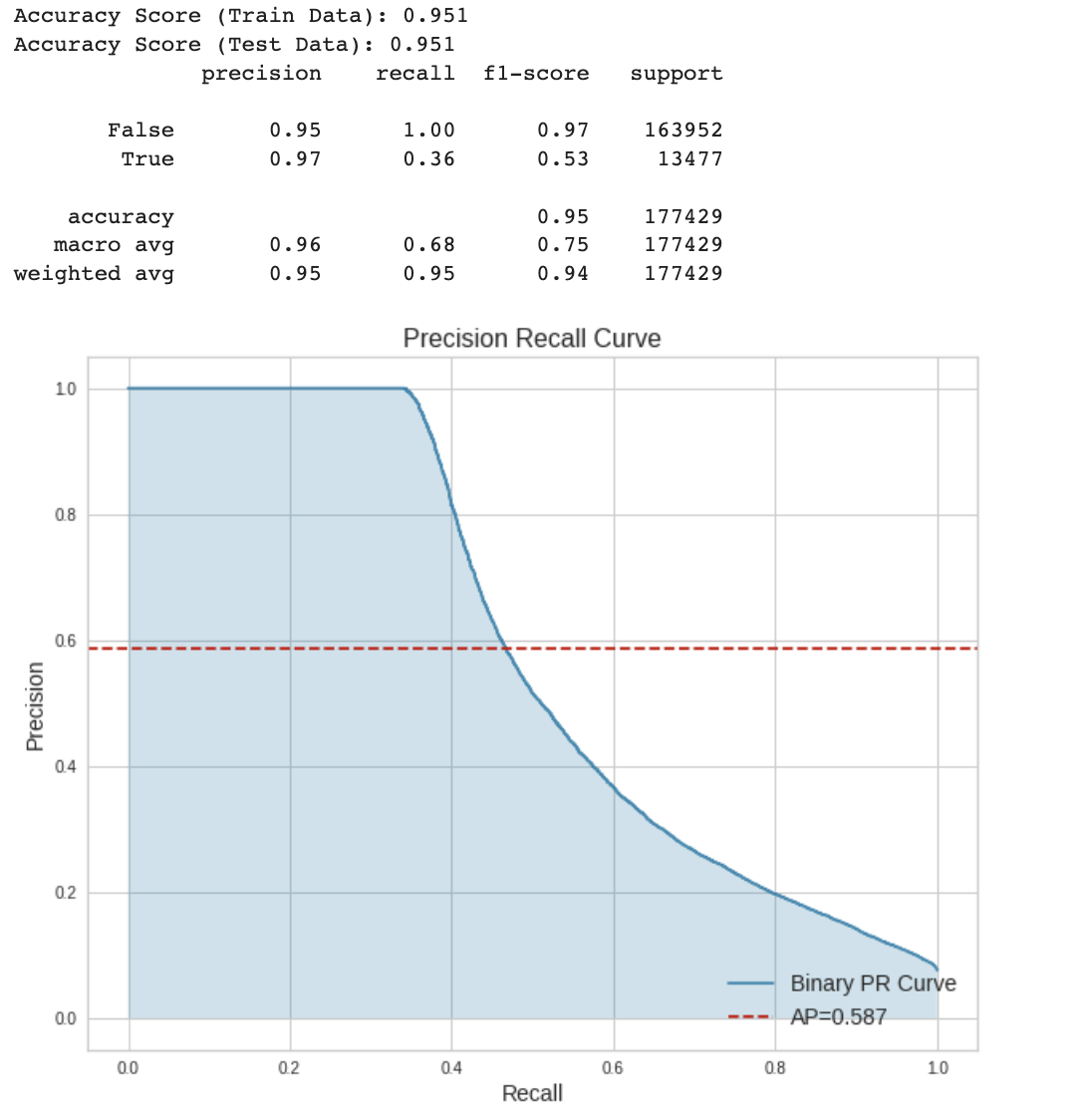In the next article, I am going to discuss Univariate, Bivariate, and Multicollinearity Analysis in Machine Learning with Examples. Here, in this article, I try to explain Logistic Regression in Machine Learning with Examples. I hope you enjoy this Logistic Regression in Machine Learning with Examples article.